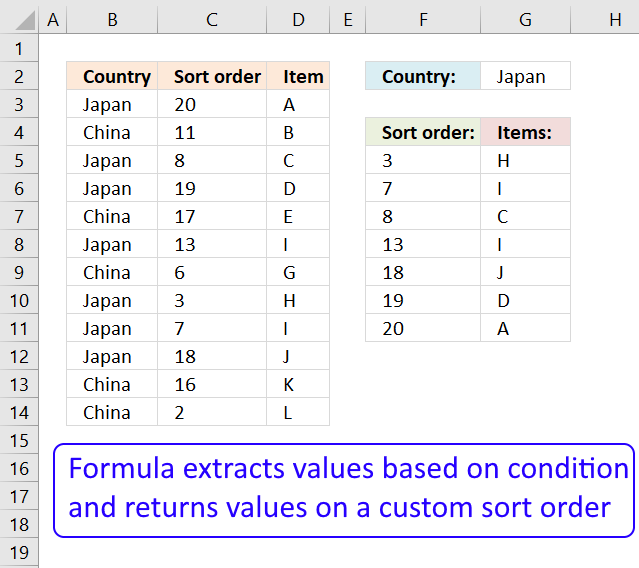Author: Oscar Cronquist Article last updated on February 19, 2018

Pat asks:

Hi Oscar,
Thanks for creating such a helpful website and I've a question if I would like to return the value with a prefix order would it possible? If not can I just add another column in the data and used it as part of the search criteria?

The array formula in cell G5 looks for the value Japan (cell G2) in column B and returns corresponding values in column D, sorted ascending by the numbers in column C.Array formula in cell G5:

=INDEX(\$D\$3:\$D\$14, MATCH(SMALL(IF(\$G\$2=\$B\$3:\$B\$14, \$C\$3:\$C\$14, ""), ROW(A1)), IF(\$G\$2=\$B\$3:\$B\$14, \$C\$3:\$C\$14, ""),0))

Array formula in cell F5:

=SMALL(IF(\$G\$2=\$B\$3:\$B\$14, \$C\$3:\$C\$14, ""),ROW(A1))

You can change the order to descending by replacing the SMALL function with the LARGE function.

How to enter an array formula

1. Copy above array formula for cell G5 (Ctrl + c)
2. Double click cell G5
3. Paste array formula (CTRL + v)
4. Press and hold CTRL + SHIFT simultaneously
5. Press Enter once
6. Release all keys

The formula now has curly brackets before and after the array formula {=array_formula},  if you did the above steps correctly.

How to copy array formula to remaining cells below

1. Select cell G5
2. Copy cell (Ctrl + c)
3. Select cell range G6:G11
4. Paste (Ctrl + v)

### Explaining array formula in cell G5

Step 1 - Filter sort numbers for selected country

IF(\$G\$2=\$B\$3:\$B\$14,\$C\$3:\$C\$14,"")

becomes

{20; ""; 8; 19; ""; 13; ""; 3; 7; 18; ""; ""}

Step 2 - Find k-th smallest value in array

SMALL(IF(\$G\$2=\$B\$3:\$B\$14, \$C\$3:\$C\$14,""), ROW(A1))

becomes

SMALL({20; ""; 8; 19; ""; 13; ""; 3; 7; 18; ""; ""}, ROW(A1))

becomes

SMALL({20; ""; 8; 19; ""; 13; ""; 3; 7; 18; ""; ""}, 1)

and returns 3.

ROW(A1) changes as we copy the cell to cells below, this makes the array formula dynamic.

Step 3 - Find the relative position of the k-th smallest value in array

MATCH(SMALL(IF(\$G\$2=\$B\$3:\$B\$14, \$C\$3:\$C\$14, ""),ROW(A1)), IF(\$G\$2=\$B\$3:\$B\$14, \$C\$3:\$C\$14, ""), 0)

becomes

MATCH(3, IF(\$G\$2=\$B\$3:\$B\$14, \$C\$3:\$C\$14, ""), 0)

becomes

MATCH(3, {20; ""; 8; 19; ""; 13; ""; 3; 7; 18; ""; ""}, 0)

and returns 8.

Step 4 - Return Item

INDEX(\$D\$3:\$D\$14, MATCH(SMALL(IF(\$G\$2=\$B\$3:\$B\$14, \$C\$3:\$C\$14, ""),ROW(A1)), IF(\$G\$2=\$B\$3:\$B\$14, \$C\$3:\$C\$14, ""), 0))

becomes

INDEX(\$D\$3:\$D\$14, 8)

becomes

INDEX({"A"; "B"; "C"; "D"; "E"; "I"; "G"; "H"; "I"; "J"; "K"; "L"}, 8)

and returns H in cell G5.

### Download excel *.xlsx file

Lookup and return multiple values sorted in a custom order.xlsx

### Functions in this post

IF(logical_test, [value_if_true], [value_if_false])
Checks whether a condition is met, and returns one value if TRUE, and another value if FALSE

SMALL(array,k)
Returns the k-th smallest number in this data set.

ROW(reference)
Returns the rownumber of a reference

INDEX(array,row_num,[column_num])
Returns a value or reference of the cell at the intersection of a particular row and column, in a given range

SMALL(array,k)
Returns the k-th smallest number in this data set.

MATCH(lookup_value, lookup_array, [match_type]
Returns the relative position of an item in an array that matches a specified value